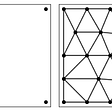# Making a simple flight simulation for model rockets in Python

Model rocketry is an awesome hobby and as a space enthusiast I constantly try to do things related to it. One such thing is making flight path simulations. I am currently working on a full scale library for flight simulations and I thought I’ll share this piece of code with everyone. So let’s get started!

# Introduction

This code is only meant for rockets that go below 1 kilometres in the air. This is because I did not want to make things really complex by adding drag into the equations. Also through out the code we will be using the SI units. I am using Python 3.7.3 but you can use any version which supports matplotlib and numpy.

# The code

Let us start off by choosing some values. I am considering the total mass with the motor (wet mass) to be 1 kg. I just randomly picked this value but if you have an actual rocket you must weigh things. Also I decided to use the Estes F15 motor because I think it is optimum for this simulation. I am going to make variables of the required properties of the motor.

`totalMass = 1dryMass = 0.906burnTime = 3.4totalImpulse = 49.6propellantMass = 0.064`

Next I am going to calculate things like the mass flow rate, the rate at which the motor uses propellant, and the average thrust. Due to simplicity, I am assuming that both of them are constant. However, this code will work even if you decide to add how those variables change with time.

`averageThrust = totalImpulse/burnTimemassFlowRate = propellantMass/burnTime`

Now I am going to make a time array that will have the different time stamps we will require. Because the burn time is to 1 decimal place, our time stamps must be to that d.p. too. However, if you want you can increase the accuracy.

`import numpy as nptime = np.linspace(0, 10, 100, False)`

“linspace” will give an array of numbers from 0 to 9.9. You can lookup the numpy documentation to learn more about linspace. So now I have a time axis.

It’s time to remember some basic physics. To get displacement we need velocity, for which we need acceleration. The area under the curve needs to be found out if we are going to find the velocity from acceleration. This is basic integration. Because this is a graph, we can easily find the area under the graph using the trapezium method. Let’s first make the array for thrust.

`index = int(np.where(time==burnTime) + 1)thrust = np.append(np.repeat(averageThrust, index), np.repeat(0, len(time) - index))`

This will make an array which has the average thrust as the values till the burn time, but after that the array only has 0. Now, we must calculate how the mass changes with time.

`mass = np.append(np.repeat(totalMass, index) - time[0:index] * massFlowRate, np.repeat(dryMass, len(time) - index))`

We did the same thing here. Now we can get the graph of acceleration by dividing the thrust array by the mass array and then subtracting 9.81 (acceleration due to gravity) from the entire array.

`acceleration = thrust/mass - 9.81`

Next let’s plot a graph of the acceleration vs the time.

`import matplotlib.pyplot as pltplt.style.use('dark_background')plt.plot(time, acceleration)plt.ylabel("Acceleration")plt.xlabel("Time")plt.show()`

This is the graph I got. You might get something different based on your intial values. The y axis is acceleration and the x axis is time.

Now it’s time to make a function that does the integration work for us. I’ll make a separate python file for this and then import the file in main.py.

I’ll call the new file “func.py”. Let’s start by importing numpy:

`import numpy as npdef integrateGraph(time, array):    pass`

For integrating, we must know the trapezium rule. Basically the area between two points in a graph is essentially the area of a trapezium. Here is an example:

If we consider the x axis as the baseline and consider 2 adjacent point, we can see that the shape formed is a trapezium. The formula is 0.5 * sum_of_parallel_sides * height. The sum of parallel sides will be the sum of the y values of the adjacent points and the height will be the time difference between those points. Doing this in a array of size 100 is tricky though. I’ll use a for loop to iterate through the array. Because the area between adjacent points will just give me the change in acceleration, I will add the add the total acceleration to the equation too.

`resArray = for n in range(0, len(time)-1):    resArray.append(        resArray[-1] + 0.5*(array[n+1] + array[n])*(time[n+1] -        time[n])    )return np.array(resArray)`

I have defaulted the initial value to 0 but that can change depending on the situation. This function will return an array with the integrated values.

In the main.py file let’s import this function and let’s use this on acceleration to calculate velocity.

`from func import integrateGraphvelocity = integrateGraph(time, acceleration)`

Let’s make the graph for velocity.

`plt.plot(time, velocity)plt.ylabel("Velocity")plt.xlabel("Time")plt.show()`

Your graph might look different but this should be the general shape. Now we can integrate the velocity graph to get the displacement graph.

`displacement = integrateGraph(time, velocity)plt.plot(time, displacement)plt.ylabel("Displacement")plt.xlabel("Time")plt.show()`

You should get a graph like this:

The moment this graph crosses the x axis it means we have hit the ground. You can plot multiple parameters over each other to analyse different things.

`plt.plot(time, displacement)plt.plot(time, velocity)plt.legend(["Displacement", "Velocity"])plt.xlabel("Time")plt.show()`

This graph shows the velocity and the displacement together. You can add labels to make the graph more understandable.

# Conclusion

So that’s it for today. This code is not very advanced and doesn’t take into account many things like drag and the launch angle. This does not mean it is not possible to add them. The launch angle for example can be taken into consideration by getting the angle from the user and multiplying the thrust array with the sin of the angle (if the angle is measured from the horizontal) or the cosine of the angle (is the angles is measured from the vertical). You can play with the code to get a better idea of your data. If you want the total change in velocity for example, instead of storing the values in an array after the integration step, you can add the values together.

I hope you enjoyed this blog! I write blogs on programming and space and if you like those topics then follow me here.

Here’s the entire code:

--

--

--

## More from Geek Culture

A new tech publication by Start it up (https://medium.com/swlh).

## MoonSwap biweekly weekly report (December 13st-December 26th)## DeckDeckGo Update: Interact With Your Audience With a Live Poll## Why Android Q is not a dessert, but a number?## Get Started with Azure Bicep — The New Azure Deployment Technology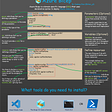## Permissions with the easiest way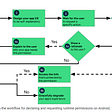## The Benefits of Feature Teams## Personal data cleaning automation project## 6 tips to Secure and Optimize your Virtual Private Server (VPS)## Tanmay Choudhary

Space exploration, AI and Flutter enthusiast. Aspiring aerospace engineer.

## The Python Planet Simulation## Triangulation with Python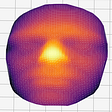## Cheating at math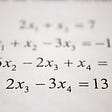## What is in the eScience toolbox: Stef on his favourite meshing library